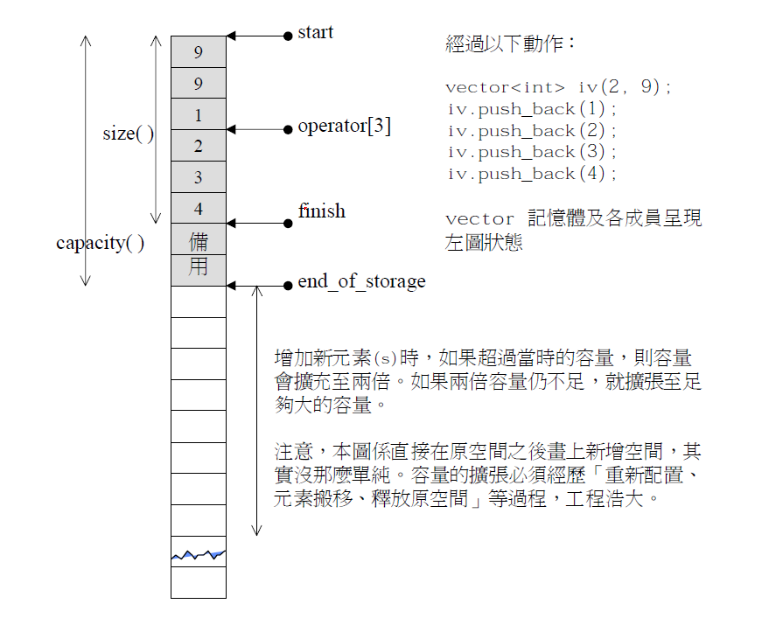# C++迭代器失效你真的理解了吗，看博主用vector的代码实现给你讲清楚迭代器失效以及解决方案！（超详细）

## vector的模拟实现

### 文章目录

• vector的模拟实现
• 1.vector的基本架构😺
• 2.重要默认成员函数🙉
• 构造函数--vector()
• 无参vector
• 让前n个空间初始化成val
• 迭代器区间初始化
• 析构函数--~vector()
• 拷贝构造
• 赋值运算符重载--operator=
• 3.三种遍历方式🐱‍👓
• 下标遍历法--operator[]
• 迭代器遍历法--begin(),end()
• 范围for遍历法
• 4.增删查改👻
• reserve()--异地增容
• reserve的一些隐患（memcpy）
• resize()
• 增--考虑增容
• push_back()--尾插
• insert()--中间插
• 删--考虑非空
• empty()
• pop_back()--尾删
• erase()--中间删
• 查改
• 5.迭代器失效问题🦝
• 什么是迭代器失效？
• insert造成的迭代器失效--迭代器失效1
• insert造成的迭代器失效的解决方法
• erase造成的迭代器失效--迭代器失效2
• erase造成的迭代器失效--迭代器失效2
• 6.vector的对应课后习题分享（题目来自leetcode，牛客网）🦄
• 7.心得分享🐣

​ 本次vector的实现顺序是：

1. 重要默认成员函数
2. 三种遍历方式（加上一些运算符重载）
3. 增删查改（核心部分）
4. 迭代器失效概念，问题，以及解决方法–重点

``````#include<iostream>
#include<vector>
#include<algorithm>
#include<assert.h>
#include<string>
#include<stdlib.h>using namespace std;
``````

### 1.vector的基本架构😺

``````template <class T>//vector的内容类型可能有int，double，string，所以咱们用模板实现
namespace kcc
{class vector{public:typedef T* iterator;typedef const T* const_iterator;private:T* _start;T* _finish;//_start + sizeT* _endofstorage;//_start + capacity};
}
``````

​ 可能稍微有点不理解的地方是，为什么基本架构里面没有了`_size` `_capacity`,其实这只是让vector的基本架构更加接近于STL的源码实现，所以将`_size``_capacity`换成了，类型的指针`_finish``_endofstorage`### 2.重要默认成员函数🙉

#### 构造函数–vector()

##### 无参vector
``````vector()
{:_start(nullptr), _finish(nullptr), _endofstorage(nullptr){}
}
``````
##### 让前n个空间初始化成val

``````vector(size_t n,const T& val = T()):_start(nullptr), _finish(nullptr), _endofstorage(nullptr)
{reserve(n);while (n--){push_back(val);}
}
``````

##### 迭代器区间初始化

``````vector(iterator first, iterator last):_start(nullptr), _finish(nullptr), _endofstorage(nullptr)
{size_t capacity= last - first;reserve(capacity);while (first != last){push_back(*first);++first;}
}
``````#### 析构函数–~vector()

``````~vector()
{//释放空间，清理指针if (_start)delete[]_start;//如果new了多个空间，delete的时候需要加[]_start = _finish = _endofstorage = nullptr;
}
``````

#### 拷贝构造

(351条消息) C++的string你可能会用，但是你模拟实现过了吗？带你实现以下string的重要接口！_龟龟不断向前的博客-CSDN博客

``````void swap(vector<T>& v)//憨逼swap怎么能加const，而且需要传引用
{::swap(_start, v._start);::swap(_finish, v._finish);::swap(_endofstorage, v._endofstorage);
}
``````
``````vector(vector<T>& v)//拷贝构造只能传引用:_start(nullptr), _finish(nullptr), _endofstorage(nullptr)
{vector<T> tmp(v.begin(), v.end());swap(tmp);
}
``````

#### 赋值运算符重载–operator=

``````vector<T>& operator=(vector<T> tmp)
{swap(tmp);return *this;
}
``````

### 3.三种遍历方式🐱‍👓

#### 下标遍历法–operator[]

``````const T& operator[](size_t i) const
{return _start[i];
}T& operator[](size_t i)
{return _start[i];
}
``````

1. 什么时候加const修饰
2. 什么时候不加const修饰
3. 什么时候既要有const修饰的，又要有非const的

``````size_t size() const//返回元素个数，即最后一个元素的下一个位置的下标
{return _finish - _start;
}size_t capacity() const//顺便也把容量这个函数也实现了
{return _endofstorage - _start;
}
``````
``````void test_vector1()
{vector<int> v1;v1.push_back(1);v1.push_back(2);v1.push_back(3);v1.push_back(4);v1.push_back(5);for (int i = 0; i < v1.size(); ++i){cout << v1[i] << " ";}cout << endl;
}
``````

#### 迭代器遍历法–begin(),end()

vector迭代器的原理我们已经实现了，就是元素的指针。

``````iterator begin()
{return _start;
}const_iterator begin() const
{return _start;
}iterator end()
{return _finish;
}const_iterator end() const
{return _finish;
}
``````
``````void test_vector2()
{vector<int> v1;v1.push_back(1);v1.push_back(2);v1.push_back(3);v1.push_back(4);v1.push_back(5);vector<int>::iterator it = v1.begin();while (it != v1.end()){*it += 1;cout << *it << " ";++it;}cout << endl;
}
``````

#### 范围for遍历法

``````void test_vector2()
{vector<int> v1;v1.push_back(1);v1.push_back(2);v1.push_back(3);v1.push_back(4);v1.push_back(5);for(auto& x: v1){cout<<x<<" ";}cout<<endl;
}
``````

### 4.增删查改👻

#### reserve()–异地增容

1. 申请一块新空间
2. 将旧空间的值拷贝到新空间
3. 将旧空间释放
``````void reserve(size_t n)
{if (n > capacity())//n大于容量才考虑增容{size_t sz = size();//要记录size的值，不然后面delete之后就找不到size了T* tmp = new T[n];for (int i = 0; i < sz; ++i){tmp[i] = _start[i];}delete _start;_start = tmp;_finish = _start + sz;_endofstorage = _start + n;}
}
``````

#### reserve的一些隐患（memcpy）

``````for (int i = 0; i < sz; ++i)
{tmp[i] = _start[i];
}
``````

`memcpy()`是按字节进行值拷贝，而string是用指针来维护的，需要深拷贝，string用`memcpy()`就会导致旧空间的内容和新空间的

#### resize()

``````void resize(size_t n ,  T val = T())
{if (n < size()){_finish = _start + n;}else{if (n > capacity()){reserve( n);}while (_finish < _start + n){*_finish = val;++_finish;}}
}
``````

#### 增–考虑增容

##### push_back()–尾插
``````void push_back(const T& val)
{if (_finish == _endofstorage)//增容{size_t newcapacity = capacity() == 0 ? 4 : capacity() * 2;//防止空vector的增容失败reserve(newcapacity);}*_finish = val;_finish++;}
``````

##### insert()–中间插
``````iterator insert(iterator pos,const T& val)//在pos的前面插入val
{assert(pos <= _finish);if (_finish == _endofstorage){size_t newcapacity = capacity() == 0 ? 4 : capacity() * 2;reserve(newcapacity);}iterator end = _finish;while ( end > pos){*end = *(end - 1);--end;}*pos = val;++_finish;return pos;
}
``````

#### 删–考虑非空

##### empty()
``````bool empty()
{return (_start == _finish);
}
``````
##### pop_back()–尾删
``````void pop_back()
{if (!empty()){--_finish;}
}
``````
##### erase()–中间删
``````iterator erase(iterator pos)//返回的是删除的元素的下一个元素{assert(pos <= _finish);iterator start = pos + 1;while (start < _finish){*(start-1) = *(start);++start;}--_finish;return pos;}
``````

### 5.迭代器失效问题🦝

#### 什么是迭代器失效？

1. 迭代器意义变了
2. vector迭代器成为野指针
##### insert造成的迭代器失效–迭代器失效1
``````void test_vector4(){vector<int> v;v.push_back(1);v.push_back(2);v.push_back(3);v.push_back(4);v.push_back(5);vector<int>::iterator pos = ::find(v.begin(), v.end(), 3);//找到3的迭代器v.insert(pos, 30);cout << *pos << endl;}
``````

##### insert造成的迭代器失效的解决方法

``````void test_vector3(){vector<int> v;v.push_back(1);v.push_back(2);v.push_back(3);v.push_back(4);v.push_back(5);vector<int>::iterator pos = ::find(v.begin(), v.end(), 3);//找到3的迭代器v.insert(pos, 30);pos = ::find(v.begin(), v.end(), 3);cout << *pos << endl;}
``````

##### erase造成的迭代器失效–迭代器失效2
``````void test_vector5(){vector<int> v;v.push_back(1);v.push_back(2);v.push_back(3);v.push_back(4);v.push_back(5);v.push_back(6);auto it = v.begin();while (it != v.end()){if (*it % 2 == 0){v.erase(it);}it++;}}
``````

1. 当it是1时，不是偶数，++it

2. 当it是2时，是偶数，进行erase操作，此时内容为1，3，4，5，6，++it，it变为4

此时我们3这个位置没有进行判断就跳过去了

3. 当it是4时，是偶数，进行erase，此时内容为1，3，5，6，++it，it变为6

此时我们5的位置又没有进行判断

4. 当it是6时，进行erase操作，此时内容为1，3，5，it也变成了end()的位置，++it

此时it成为野指针，迭代器失效

所以这段程序即有内容变了失效，又有成为野指针失效。

1. vs编译器对迭代器失效有检查，当迭代器效率的时候，对齐进行++，–，*是会报错的，所以还是无法解决问题
2. 其次，vector迭代器是因为空间的连续性，下一个元素在erase操作之后正好落在了it上。但是链表可不会这样了

##### erase造成的迭代器失效–迭代器失效2

``````void test_vector5(){vector<int> v;v.push_back(1);v.push_back(2);v.push_back(3);v.push_back(4);v.push_back(5);v.push_back(6);auto it = v.begin();while (it != v.end()){if (*it % 2 == 0){it = v.erase(it);}else{++it;}}}
``````

1. insert
2. erase
3. 包括reserve异地增容，内存位置发生变化，pos成为野指针
4. 异地缩容也会

### 6.vector的对应课后习题分享（题目来自leetcode，牛客网）🦄

1. 136. 只出现一次的数字 - 力扣（LeetCode）
2. 118. 杨辉三角 - 力扣（LeetCode）
3. 26. 删除有序数组中的重复项 - 力扣（LeetCode）
4. 137. 只出现一次的数字 II - 力扣（LeetCode）
5. 数组中出现次数超过一半的数字_牛客题霸_牛客网 (nowcoder.com)
6. 260. 只出现一次的数字 III - 力扣（LeetCode）
7. 17. 电话号码的字母组合 - 力扣（LeetCode）
8. 连续子数组的最大和_牛客题霸_牛客网 (nowcoder.com)

### 7.心得分享🐣

​ 最近还是挺迷的，而且特别不想写博客，因为想到博客就会去想写一篇博客至少得花两三个小时，真的很浪费时间，但是想了想如果写了博客：

1. 之后的复习会很方便
2. 对只是点的消化也会更好
3. 也时一种记录学习的方式